### Descriptive Statistics for One Quantitative Variable

There are three basic questions you need to answer to explore a single quantitative variable.

1. Location - basically, where is the data located?
2. Variation (scatter, spread, etc.) - how spread out is the data?
3. Shape - Beyond location and variation, what should be said about the data?

Let’s create some artificial data to explore these ideas

``x = rnorm(1000)``

That created a vector x with 1,000 numbers drawn from a normal distribution with mean = 0 and standard deviation = 1. Note that nothing seems to have happened. It is worth remembering that in R creating something doesn’t automatically display it. But we can do many things to explore x.

Look at its actual measures of location and variation to see if they are close to what we would expect from the way we created x.

``mean(x)``
``##  -0.01732378``
``median(x)``
``##  -0.02207803``
``sd(x)``
``##  0.9823172``
``range(x)``
``##  -2.738547  2.843015``
``max(x) - min(x)``
``##  5.581562``
``IQR(x)``
``##  1.330111``

### Following Changes

Why do we call something a measure of location or variation of a collection of numbers? In a practical sense, we want the measure to reflect changes that we make to the set of numbers. If we move the set of numbers to the right, we want the measure to move to the right. If we spread the numbers out more, we want the measure of variation to increase

Now let’s change x and see what happens. First add 100 to each number in the vector

``xplus100 = x + 100``

Now look at our measures of the new version of x.

``mean(xplus100)``
``##  99.98268``
``median(xplus100)``
``##  99.97792``
``sd(xplus100)``
``##  0.9823172``
``range(xplus100)``
``##   97.26145 102.84301``
``max(xplus100) - min(xplus100)``
``##  5.581562``
``IQR(xplus100)``
``##  1.330111``

This should have changed the location measures, increasing them by 100, but it should leave the variation nummbers alone. Is this what happened?

What about graphical displays of x? The standard displays are the histogram and the boxplot.

Now let’s multiply x by 100 and see what happens to our measures of location and variation.

``````xtimes100 = x * 100
mean(xtimes100)``````
``##  -1.732378``
``median(xtimes100)``
``##  -2.207803``
``sd(xtimes100)``
``##  98.23172``
``range(xtimes100)``
``##  -273.8547  284.3015``
``max(xtimes100) - min(xtimes100)``
``##  558.1562``
``IQR(xtimes100)``
``##  133.0111``

I’ll leave it as an exercise for you to describe the relationships between the measures of location and variation for x and those for xtimes100.

### Shape - Graphical Displays

The standard graphical displays for quantitative variables are the histogram and the boxplot. To be comparable with the histogram, you may want to ask that the boxplot be laid out horizontally instead of vertically, which is the default. There is a command summary(), which produces the key numerical results displayed in the boxplot.

``hist(x)``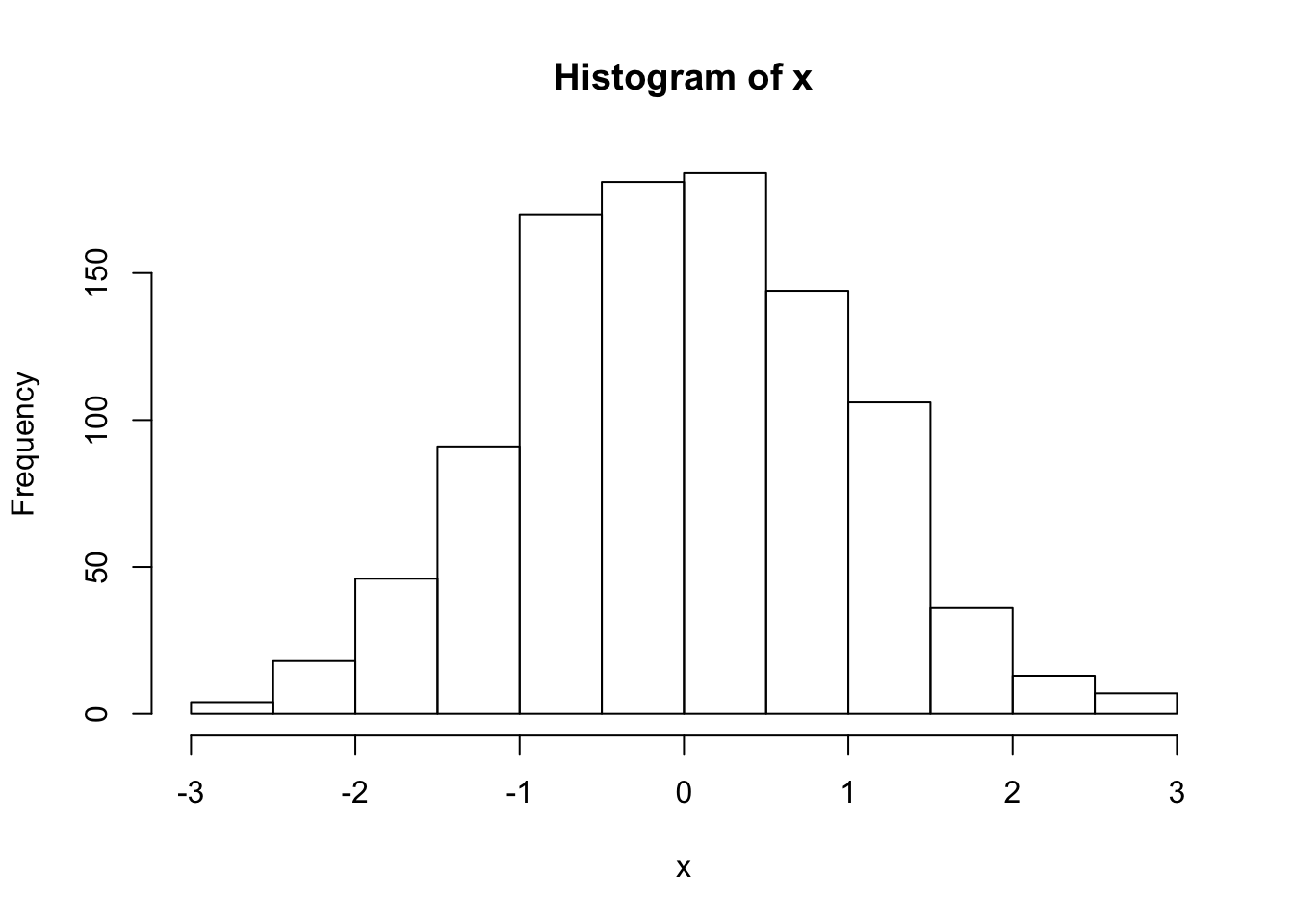``boxplot(x,horizontal=TRUE)``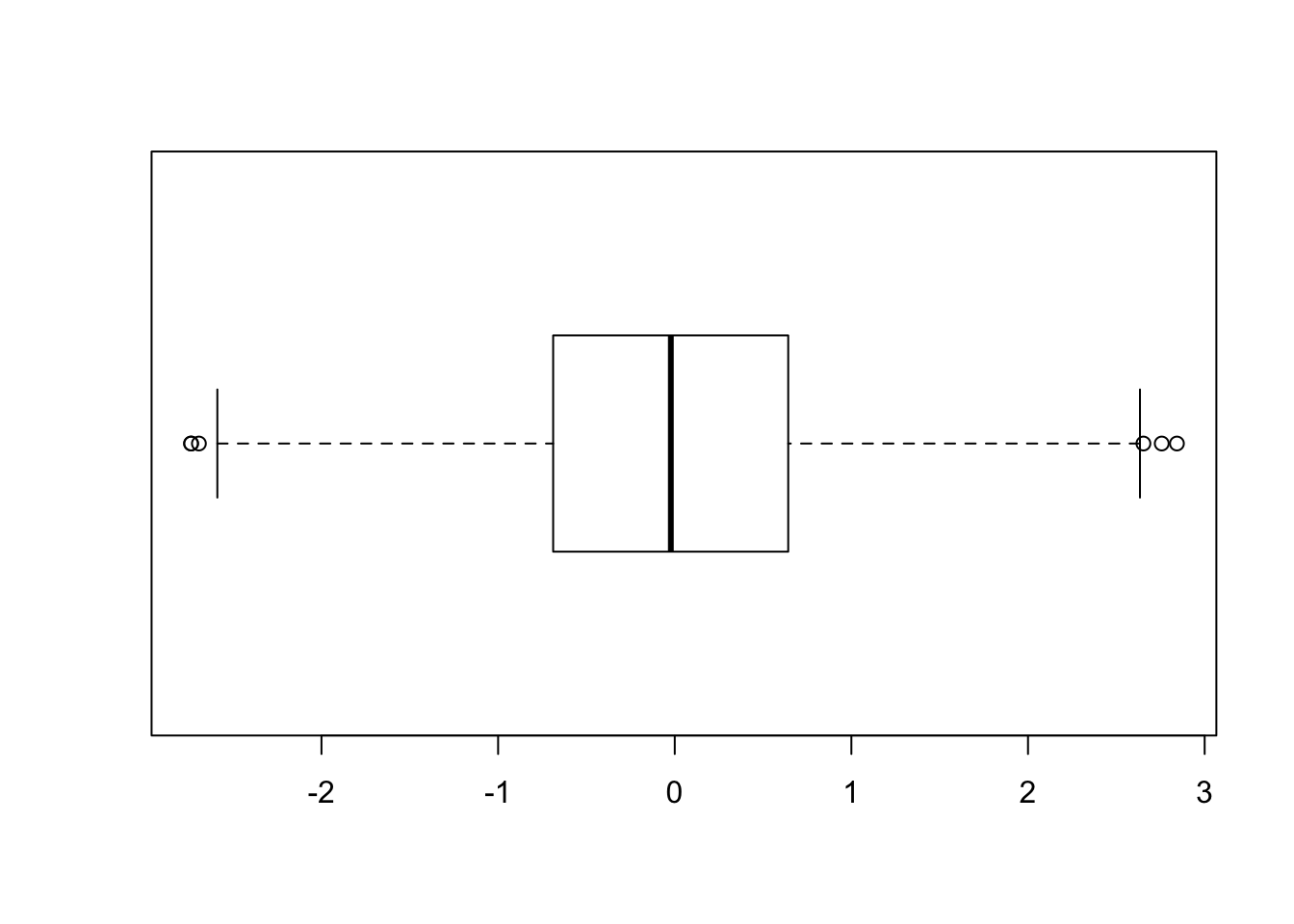``summary(x)``
``````##     Min.  1st Qu.   Median     Mean  3rd Qu.     Max.
## -2.73900 -0.68830 -0.02208 -0.01732  0.64180  2.84300``````

These graphical displays of x show a very conventional symmetric distribution with a single central peak. It is useful to look at some other examples to see a few possibilities. Let’s generate some uniformly distributed numbers between 5 and 10 and create the graphical displays.

``````flatones = runif(1000,min=5,max=10)
summary(flatones)``````
``````##    Min. 1st Qu.  Median    Mean 3rd Qu.    Max.
##   5.021   6.347   7.524   7.547   8.819   9.994``````
``boxplot(flatones,horizontal=TRUE)``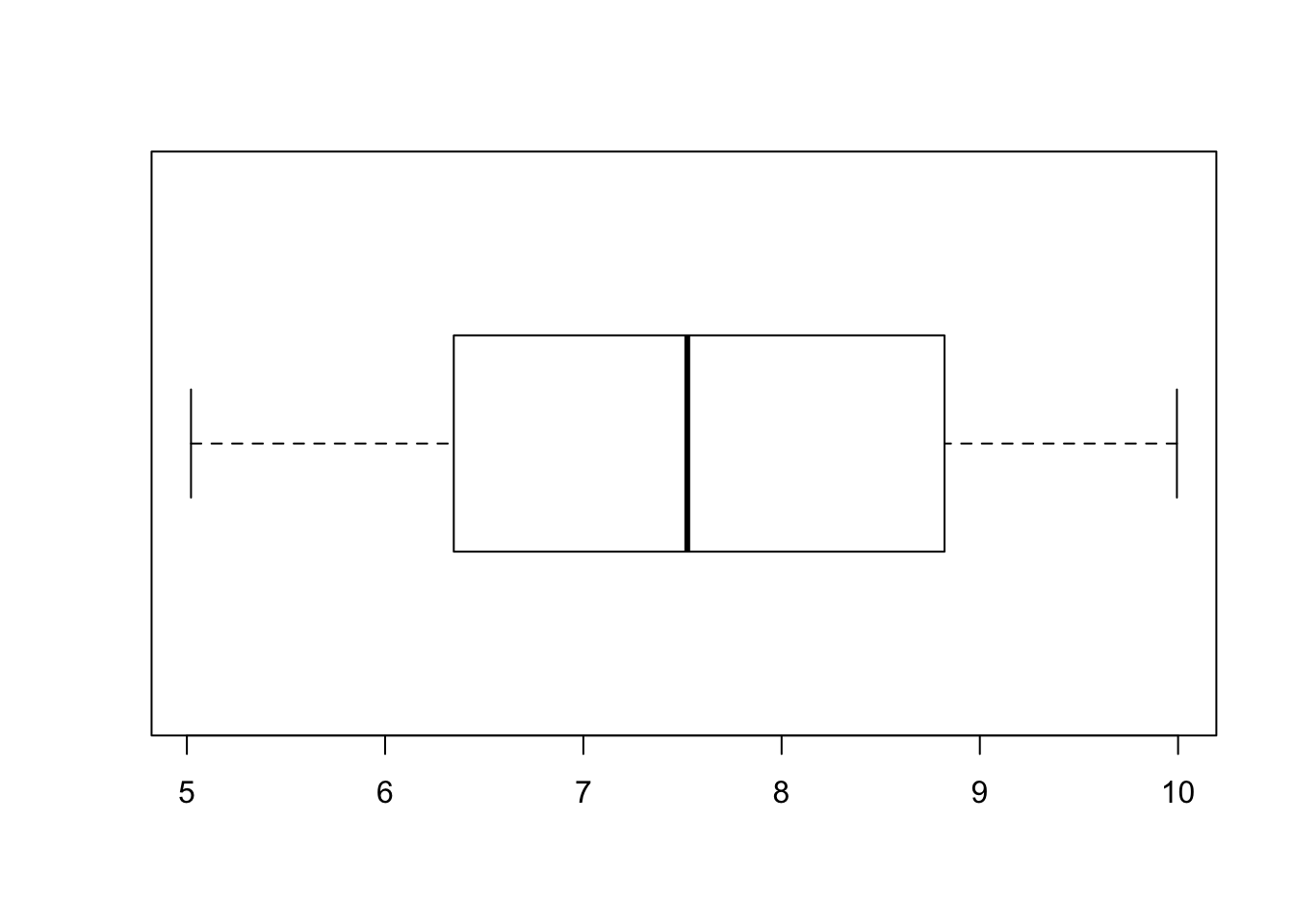``hist(flatones)``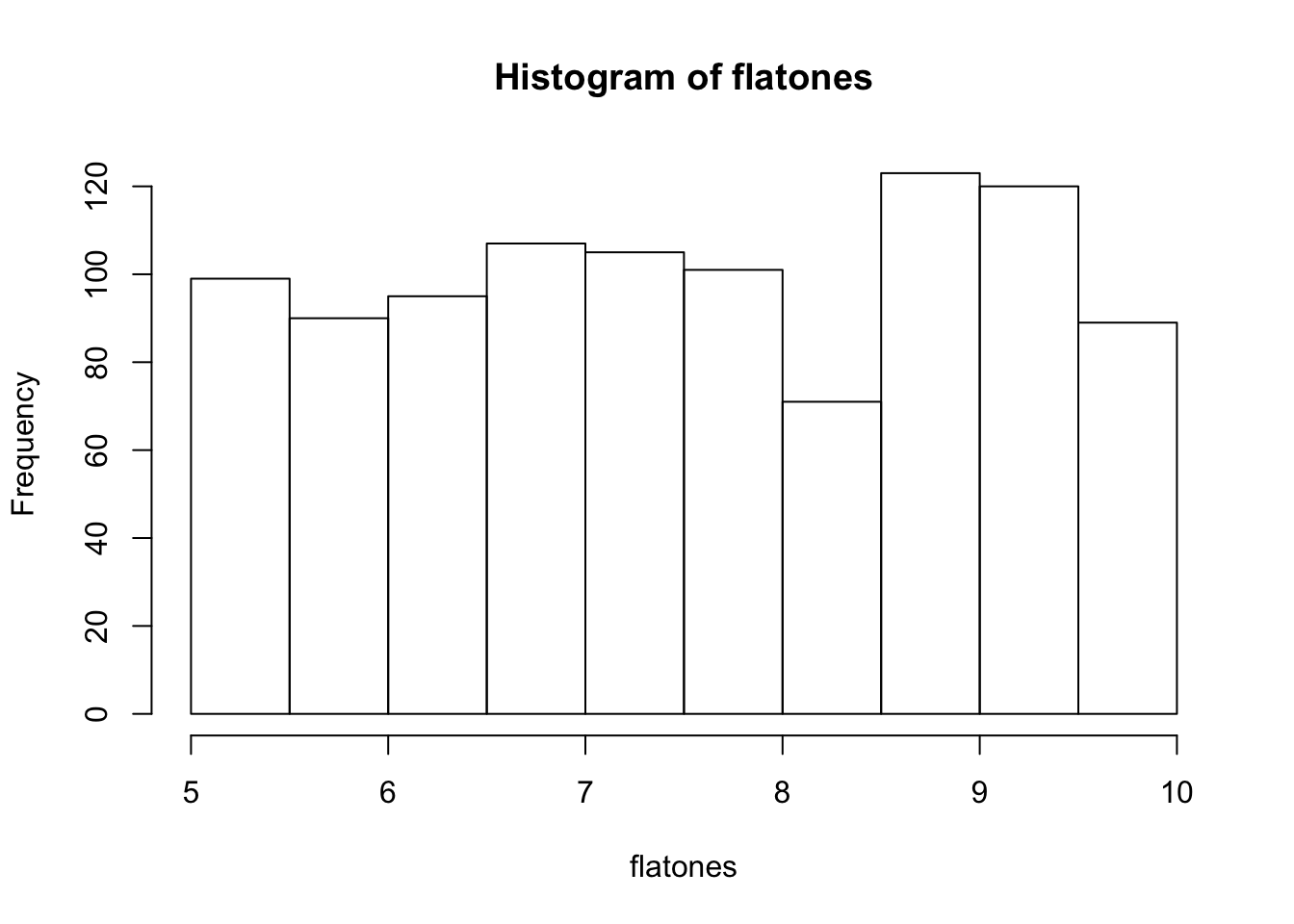Do you see what you expected to see. Is this distribution symmetric? is there a noticeable peak? Are there outliers?

Let’s try some values drawn from a Chi-squared distribution with 10 degrees of freedom. Don’t worry about what this means. Just concentrate on the shape questions.

``````cq = rchisq(1000,df=10)
hist(cq)``````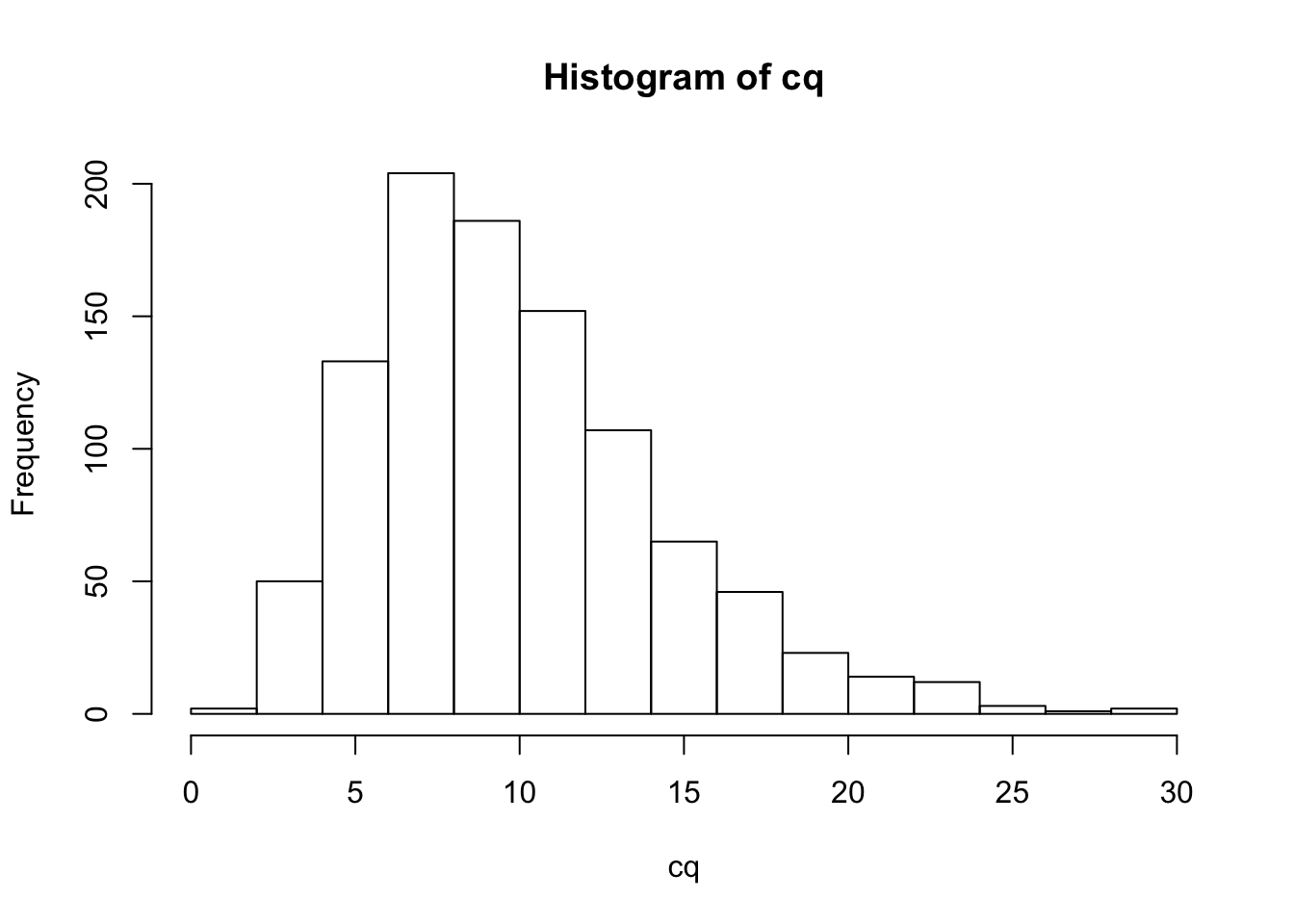``boxplot(cq,horizontal=TRUE)``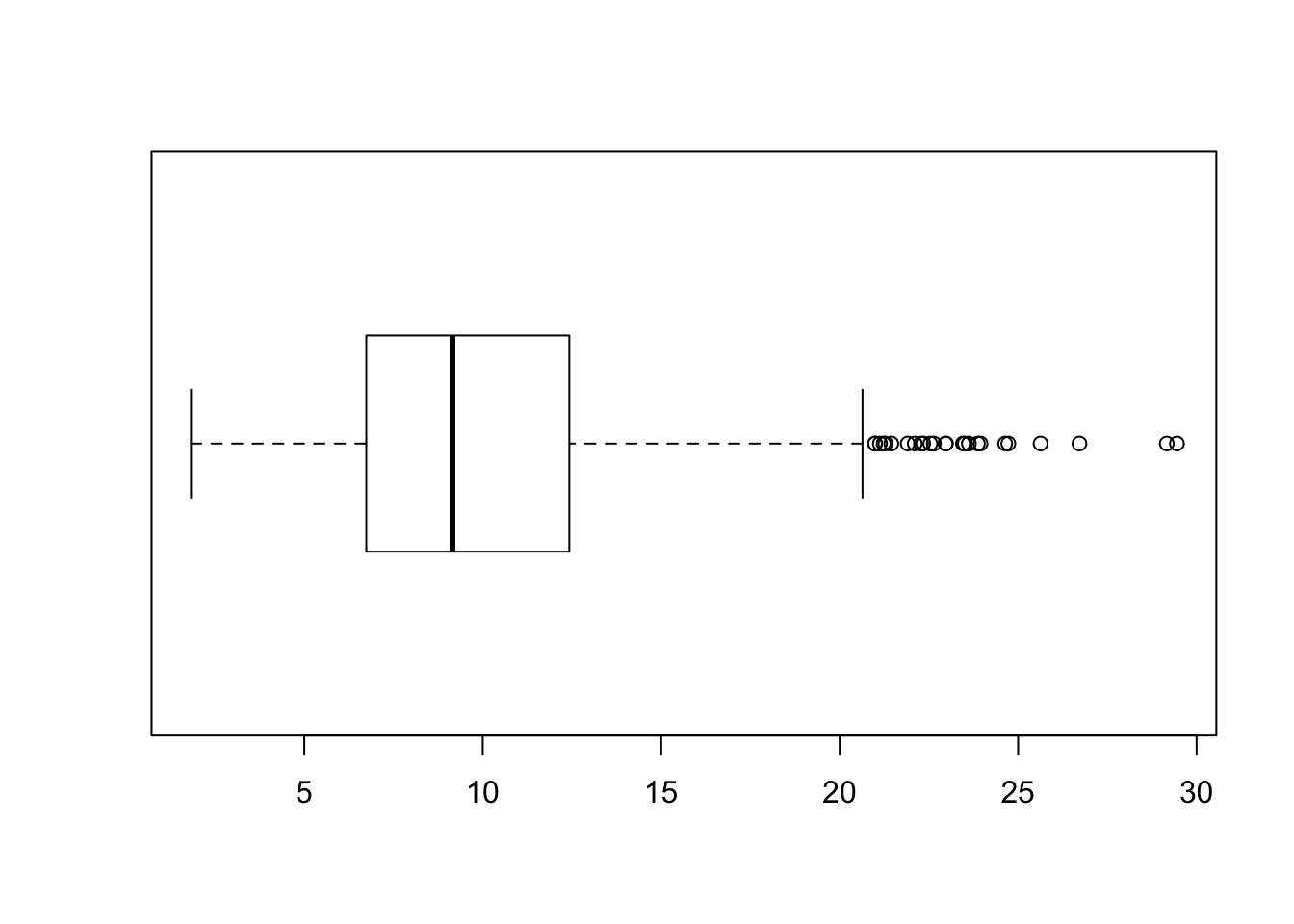Is this distribution symmetric? Is there a noticeable peak? Are there outliers?

### Descriptive Statistics for one Categorical Variable

We’ll look at the mtcars dataset, which is included with the base distribution of R as a dataframe. First we’ll run a few standard commands to examine a new dataframe when we know nothing but the name of the dataframe.

``str(mtcars)``
``````## 'data.frame':    32 obs. of  11 variables:
##  \$ mpg : num  21 21 22.8 21.4 18.7 18.1 14.3 24.4 22.8 19.2 ...
##  \$ cyl : num  6 6 4 6 8 6 8 4 4 6 ...
##  \$ disp: num  160 160 108 258 360 ...
##  \$ hp  : num  110 110 93 110 175 105 245 62 95 123 ...
##  \$ drat: num  3.9 3.9 3.85 3.08 3.15 2.76 3.21 3.69 3.92 3.92 ...
##  \$ wt  : num  2.62 2.88 2.32 3.21 3.44 ...
##  \$ qsec: num  16.5 17 18.6 19.4 17 ...
##  \$ vs  : num  0 0 1 1 0 1 0 1 1 1 ...
##  \$ am  : num  1 1 1 0 0 0 0 0 0 0 ...
##  \$ gear: num  4 4 4 3 3 3 3 4 4 4 ...
##  \$ carb: num  4 4 1 1 2 1 4 2 2 4 ...``````
``summary(mtcars)``
``````##       mpg             cyl             disp             hp
##  Min.   :10.40   Min.   :4.000   Min.   : 71.1   Min.   : 52.0
##  1st Qu.:15.43   1st Qu.:4.000   1st Qu.:120.8   1st Qu.: 96.5
##  Median :19.20   Median :6.000   Median :196.3   Median :123.0
##  Mean   :20.09   Mean   :6.188   Mean   :230.7   Mean   :146.7
##  3rd Qu.:22.80   3rd Qu.:8.000   3rd Qu.:326.0   3rd Qu.:180.0
##  Max.   :33.90   Max.   :8.000   Max.   :472.0   Max.   :335.0
##       drat             wt             qsec             vs
##  Min.   :2.760   Min.   :1.513   Min.   :14.50   Min.   :0.0000
##  1st Qu.:3.080   1st Qu.:2.581   1st Qu.:16.89   1st Qu.:0.0000
##  Median :3.695   Median :3.325   Median :17.71   Median :0.0000
##  Mean   :3.597   Mean   :3.217   Mean   :17.85   Mean   :0.4375
##  3rd Qu.:3.920   3rd Qu.:3.610   3rd Qu.:18.90   3rd Qu.:1.0000
##  Max.   :4.930   Max.   :5.424   Max.   :22.90   Max.   :1.0000
##        am              gear            carb
##  Min.   :0.0000   Min.   :3.000   Min.   :1.000
##  1st Qu.:0.0000   1st Qu.:3.000   1st Qu.:2.000
##  Median :0.0000   Median :4.000   Median :2.000
##  Mean   :0.4062   Mean   :3.688   Mean   :2.812
##  3rd Qu.:1.0000   3rd Qu.:4.000   3rd Qu.:4.000
##  Max.   :1.0000   Max.   :5.000   Max.   :8.000``````

Note that there are some numerical variables here, which are categorical in nature. One example is ‘am,’ which tells us whether the car has an automatic (am = 0) or manual transmission (am = 1). To create a variable that R will treat as categorical, we need to run a special command.

``TranType = as.factor(mtcars\$am)``

Now we can run the standard commands to exploare a categorical variable.

``````# Get simple counts of each categorical value
table(TranType)``````
``````## TranType
##  0  1
## 19 13``````
``````# get proportions of each categorical value
table(TranType)/length(TranType)``````
``````## TranType
##       0       1
## 0.59375 0.40625``````
``````# Create a barplot of the values
barplot(table(TranType))``````